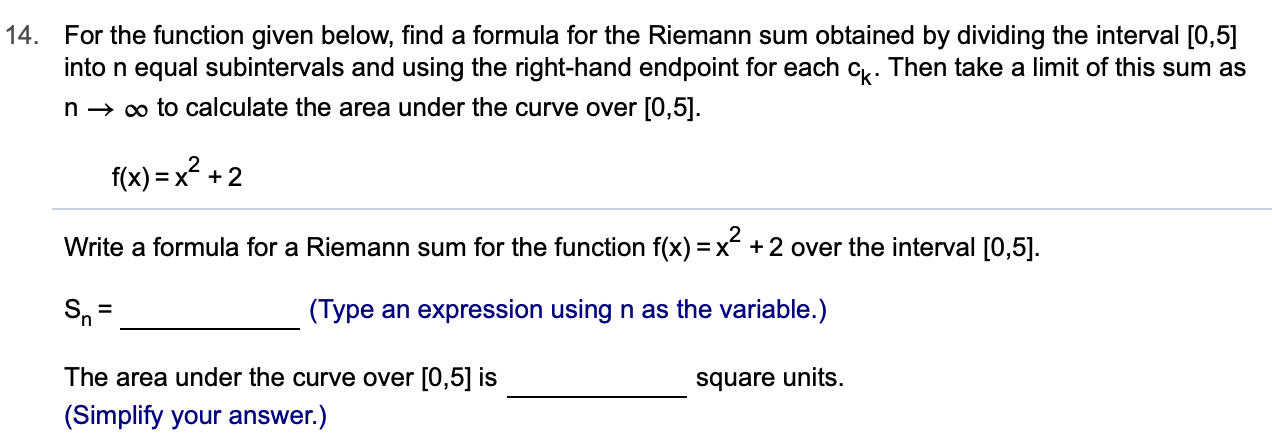# 14.For the function given below, find a formula for the Riemann sum obtained by dividing the interval [0,5]into n equal subintervals and using the right-hand endpoint for each c. Then take a limit of this sum asn oo to calculate the area under the curve over [0,5]f(x)x+2Write a formula for a Riemann sum for the function f(x) = x- +2 over the interval [0,5].(Type an expression using n as the variable.)The area under the curve over [0,5] issquare units(Simplify your answer.)

Question
2 views

Can i get help step by step with this problem?help_outlineImage Transcriptionclose14. For the function given below, find a formula for the Riemann sum obtained by dividing the interval [0,5] into n equal subintervals and using the right-hand endpoint for each c. Then take a limit of this sum as n oo to calculate the area under the curve over [0,5] f(x)x+2 Write a formula for a Riemann sum for the function f(x) = x- +2 over the interval [0,5]. (Type an expression using n as the variable.) The area under the curve over [0,5] is square units (Simplify your answer.) fullscreen
check_circle

Step 1

a=0 and b=5

So, width of each interval = 5/n

Step 2

Right end point of c_k interval is 5k/n

Right end points are given by 5/n, 10/n , 15/n , ......, 5

Step 3

So the right Riemann sum is given by: sum of f(x_k)*del x

Where x_...

### Want to see the full answer?

See Solution

#### Want to see this answer and more?

Solutions are written by subject experts who are available 24/7. Questions are typically answered within 1 hour.*

See Solution
*Response times may vary by subject and question.
Tagged in

### Other